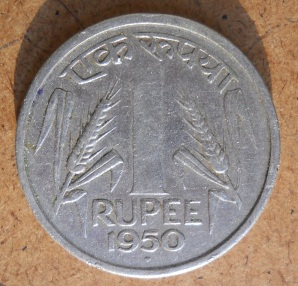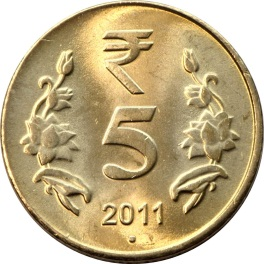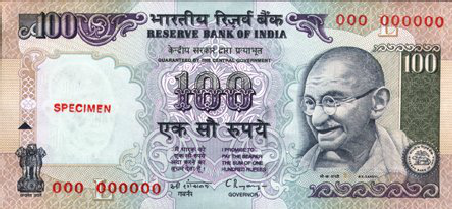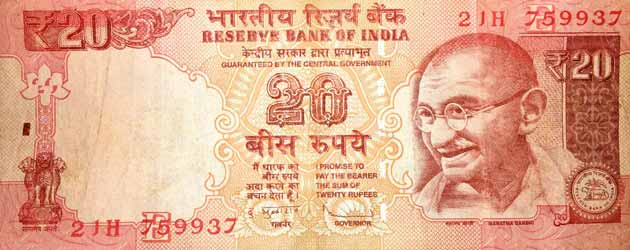## MONEY - ESSENTIAL POINTS

We need money to buy things. We need money even to buy the toffees.  The money is to be paid according to its price, when we buy things.

1. The money is in coins and in notes.

1. 1 RUPEE COIN2. 5 RUPEES COIN3. 100 RUPEES NOTE4. 20 RUPEES NOTE2. If you want to make 5 rupees, then it can be made of five one rupee coins,  1 + 1 + 1 + 1 + 1 = 5

3. It can also be made from two 2 rupees coin and one rupee coin , 2 + 2 + 1 = 5

4. If you want to make 8 rupees then it can be made from one 2 rupee coins and the rest one rupee coins like this 2 + 1 + 1 + 1 + 1 + 1 + 1 = 8

5. It can also be made from five rupee coins, one 1 rupee coin and one 2 rupees coin , 5 + 1 + 2 = 8

6. If we have to purchase the chocolate which is of Rs 40 and we have only Rs 30, then we cannot purchase that chocolate. But if we have a note of Rs 50, then we can purchase that chocolate.

7. Rs 20 can be exchanged with 4 notes of Rs 5 each OR  10 coins of Rs 2 each OR 20 coins of Re 1 each.

8. If the cost of the toy car is Rs 87 and we have only Rs 60, then we need Rs 27 more to purchase it.

9. If the cost of one duster is Rs 20, then the cost of three dusters is Rs 60. ( 20 X 3 )